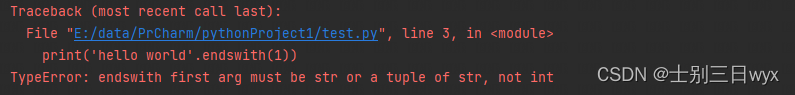##MSIPO技术圈 首页 IT技术 查看内容

# Python endswith()函数使用详解

2023-07-13

「作者主页」：士别三日wyx
「作者简介」：CSDN top100、阿里云博客专家、华为云享专家、网络安全领域优质创作者
「推荐专栏」：小白零基础《Python入门到精通》

### endswith函数使用详解

endwith() 可以「判断」字符串是否以指定内容「结尾」

``````string.endwith( str, start, end )
``````

• str ：（必选，字符串或元组）指定字符串或元素
• start ：（可选）开始的索引，默认值0
• end ：（可选）结束的索引，默认值-1

• 以指定内容结尾返回 True
• 不以指定内容结尾返回 False

``````print('hello world'.endswith('world'))
``````

``````True
``````

# 1、指定范围

``````print('hello world'.endswith('world', 1))
``````

``````True
``````

``````print('hello world'.endswith('world', 0, 5))
``````

``````False
``````

``````print('hello world'[0:5].endswith('world'))
``````

``````False
``````

# 2、str可以传入元组

str 参数只能是字符串或者元祖（元素都是字符串类型），否则会报错 TypeError: endswith first arg must be str or a tuple of str``````print('hello world'.endswith(('world', 'a')))
print('hello world'.endswith(('b', 'a')))
``````

``````True
False
``````

``````file_name = 'shell.php'
if file_name.endswith(('php', 'jsp', 'asp')):
print('被禁止的文件类型，请重新上传')
else:
print('上传成功')
``````

``````list1 = ['world', 'a', 'b']
tuple1 = tuple(list1)
print('hello world'.endswith(tuple1))
``````

``````True
``````

# 3、空字符串为真

``````print('hello world'.endswith(''))
print('*&ab31'.endswith(''))
``````

``````True
True
``````

「空格」就不行了，会返回False

``````print('hello world'.endswith(' '))
``````

``````False
``````

# 4、大小写敏感

endwith() 判断时，区分「大小写」，这导致我们匹配不到一些文件后缀名，比如下面这样会返回False

``````file_name = 'shell.PHP'
print(file_name.endswith('php'))
``````

``````False
``````

``````file_name = 'shell.pHp'
print(file_name.lower().endswith('php'))
``````

``````True
``````